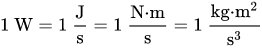# Watts to VA free online calculator

Watts (W) to volt-amps (VA) calculator.

Enter real power in watts and power factor and press the Calculate button to get the apparent power in volt-amps:

W
VA

VA to watts calculator »

## Watts to VA calculation

The apparent power S in volt-amps (VA) is equal to the real power P in watts (W), divided by the power factor PF:

S(VA) =  P(W) / PF

## Watt unit definition

The watt (symbol: W) is a unit of power or radiant flux. In the International System of Units (SI), it is defined as a derived unit of (in SI base units) 1 kg⋅m2⋅s−3 or, equivalently, 1 joule per second. It is used to quantify the rate of energy transfer. The watt is named after James Watt, an 18th-century Scottish inventor.

When an object's velocity is held constant at one metre per second against a constant opposing force of one newton, the rate at which work is done is one watt.In terms of electromagnetism, one watt is the rate at which electrical work is performed when a current of one ampere (A) flows across an electrical potential difference of one volt (V), meaning the watt is equivalent to the volt-ampere (the latter unit, however, is used for a different quantity from the real power of an electrical circuit).

1 W = 1 V * 1 A

Two additional unit conversions for watt can be found using the above equation and Ohm's law.

1 W = 1 / (V*V/Ω)

where ohm (Ω) is the SI derived unit of electrical resistance.

## Volt-ampere (VA) definition

A volt-ampere (SI symbol: V⋅A or V A; also VA) is the unit used for the apparent power in an electrical circuit. The apparent power equals the product of root mean square voltage and root mean square current. In direct current (DC) circuits, this product is equal to the real power in watts. Volt-amperes are usually used for analyzing alternating current (AC) circuits. The volt-ampere is dimensionally equivalent to the watt (in SI units, 1 V⋅A = 1 W). VA rating is most useful in rating wires and switches (and other power handling equipment) for inductive loads

For a simple electrical circuit running on direct current, the electrical current and voltage are constant. In that case, the real power (P, measured in watts) is the product of the electrical current (I, measured in amperes) and the voltage from one side of the circuit to the other (V, measured in volts):

P = I × V

However, for alternating current, both the voltage and current fluctuate over time. The apparent power (S, measured in volt-amperes) is calculated using the root mean square voltage (Vrms, measured in volts) and root mean square current (Irms measured in amps):

S = Irms × Vrms

VA to watts calculator »

Currently, we have around 1975 calculators, conversion tables and usefull online tools and software features for students, teaching and teachers, designers and simply for everyone.